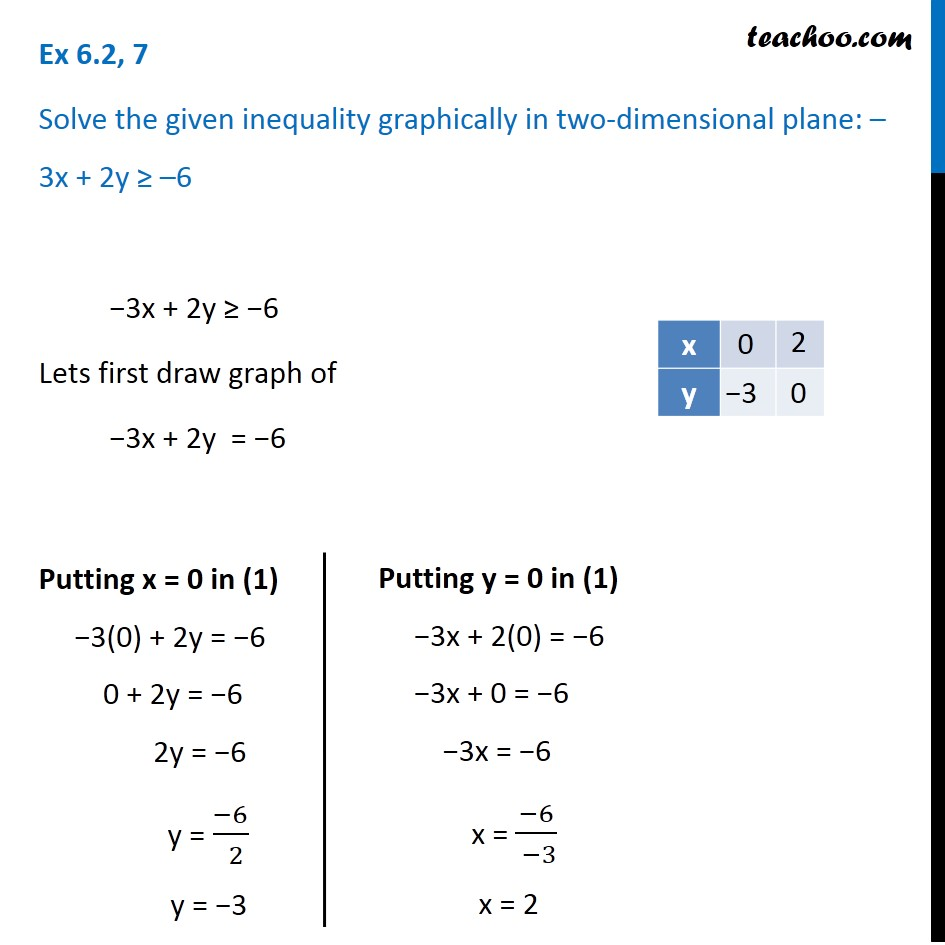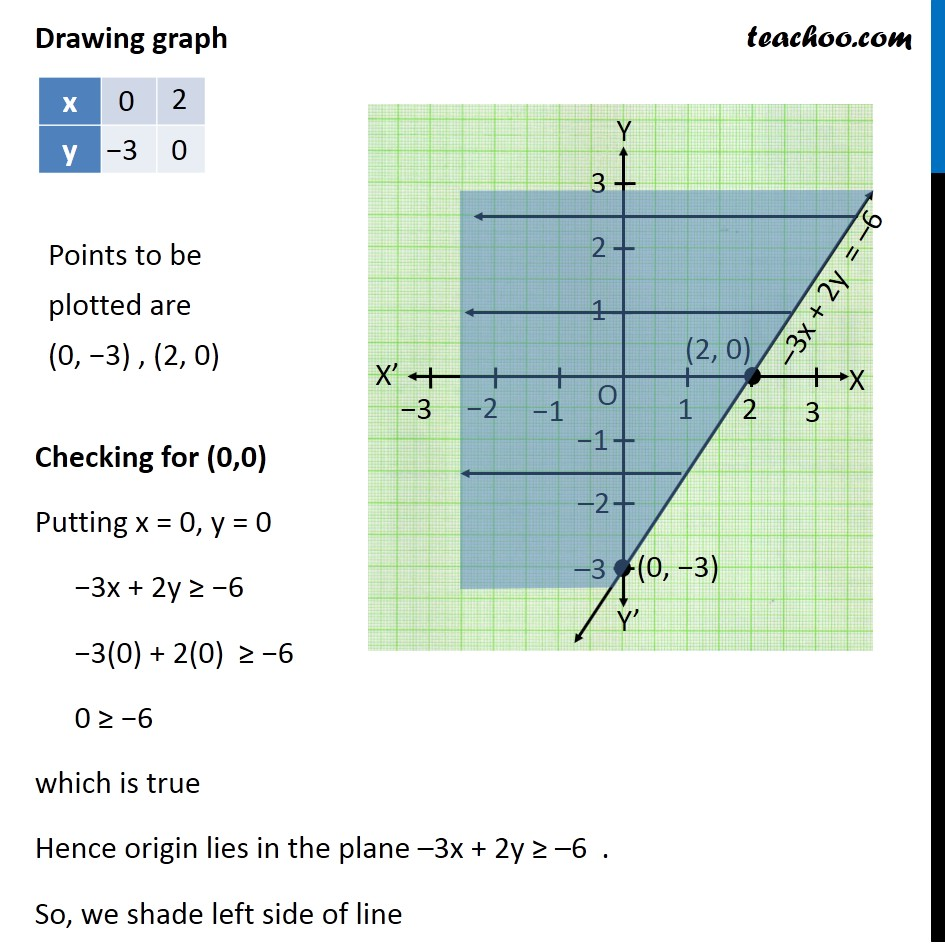Solving Linear Inequality Graphically

Chapter 5 Class 11 Linear Inequalities
Serial order wiseLearn in your speed, with individual attention - Teachoo Maths 1-on-1 Class

### Transcript

Question 7 Solve the given inequality graphically in two-dimensional plane: –3x + 2y ≥ –6 −3x + 2y ≥ −6 Lets first draw graph of −3x + 2y = −6 Putting x = 0 in (1) −3(0) + 2y = −6 0 + 2y = −6 2y = −6 y = (−6)/( 2) y = −3 Putting y = 0 in (1) −3x + 2(0) = −6 −3x + 0 = −6 −3x = −6 x = (−6)/( −3) x = 2 Drawing graph Checking for (0,0) Putting x = 0, y = 0 −3x + 2y ≥ −6 −3(0) + 2(0) ≥ −6 0 ≥ −6 which is true Hence origin lies in the plane –3x + 2y ≥ –6 . So, we shade left side of line